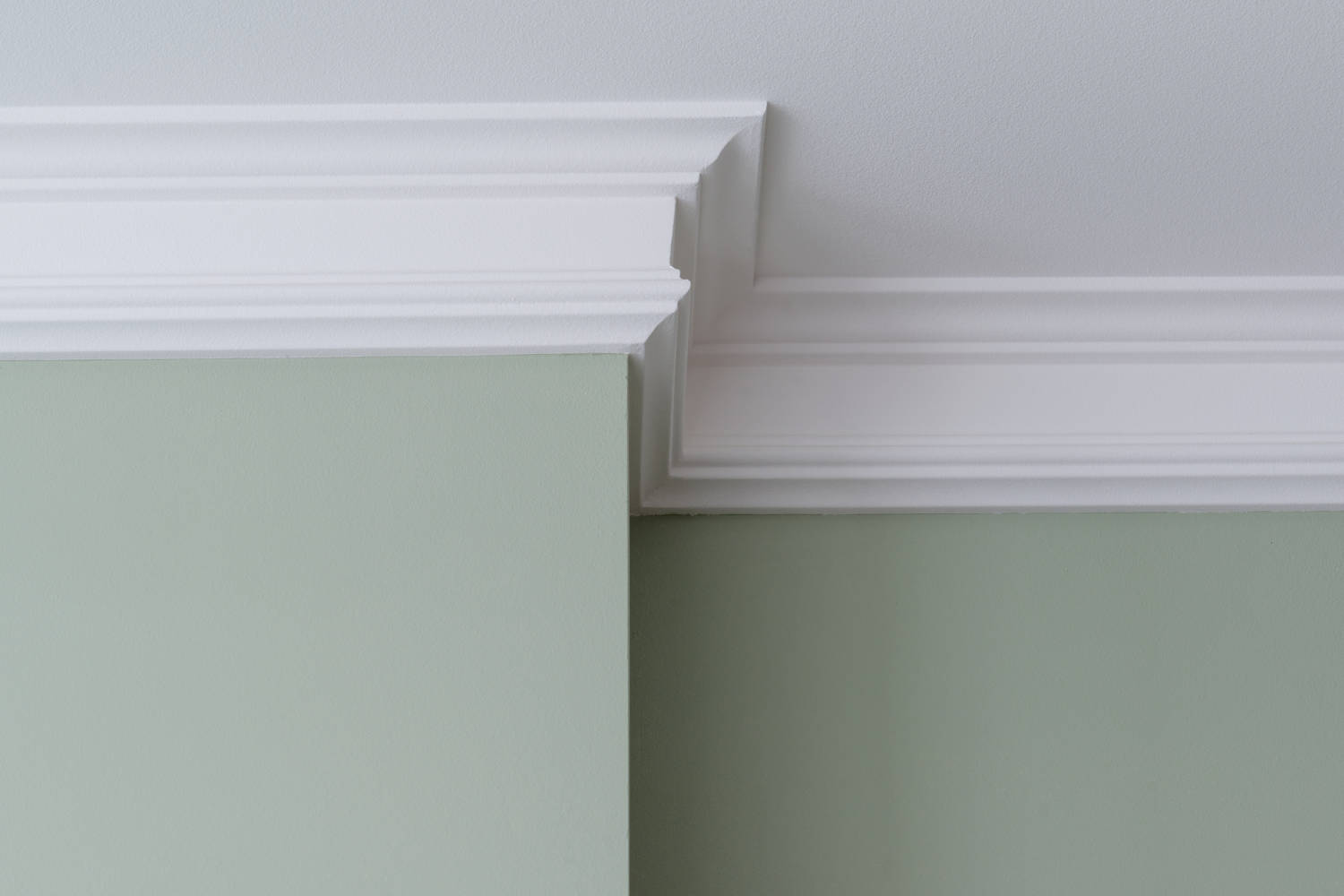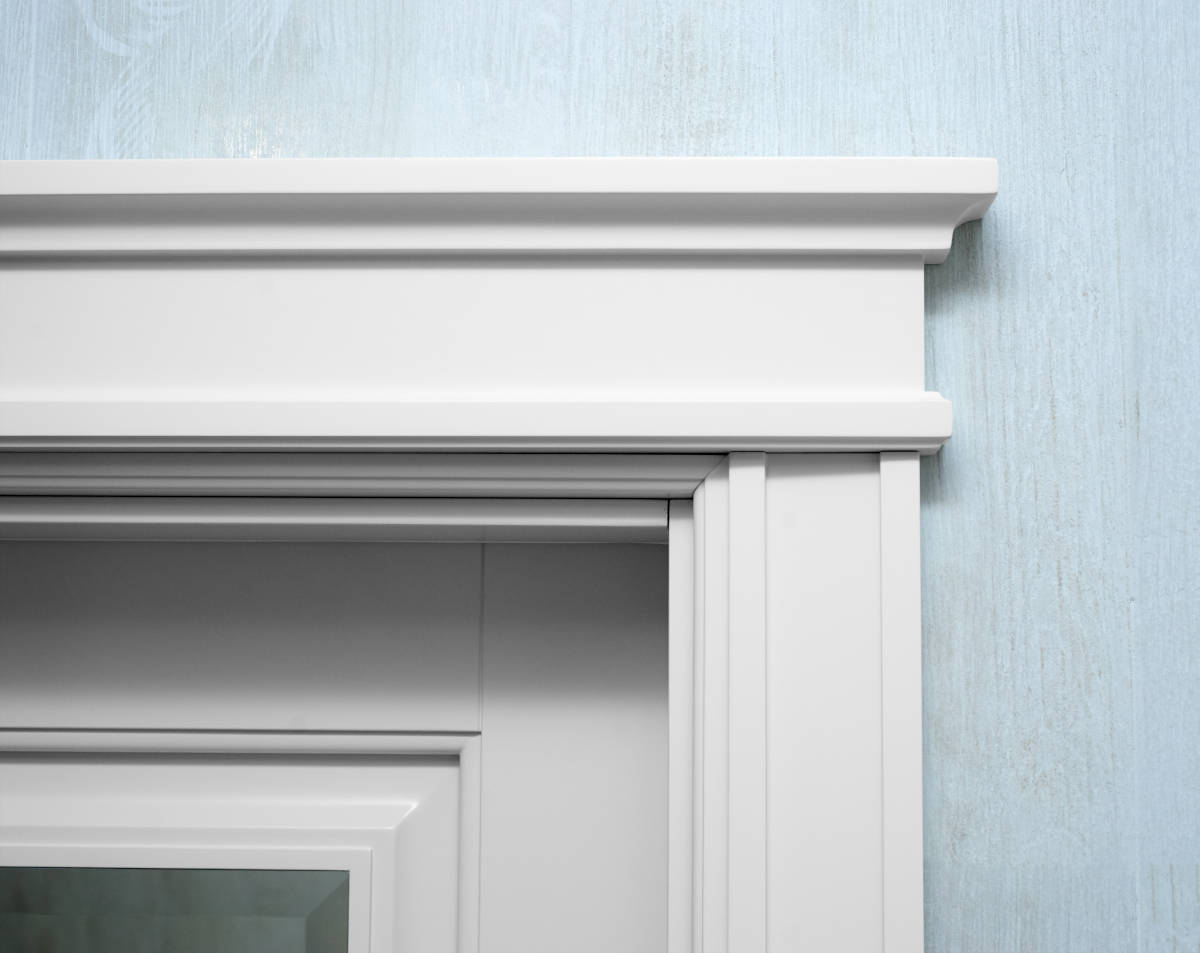# Trim and Moulding Calculator

Estimate the number of base trim, door casing, window casing, crown, and chair rail mouldings needed to complete a room by entering the length and width of your room. Optionally enter the number of windows and doors to estimate the amount of casings needed.

*Door casing estimates assume 32" x 80" doors. Window casing estimates assume 32" x 54" windows, for larger windows multiply the amount of casing needed by 2 or 3.

## Material Estimate:

Base Trim

8' mouldings

linear feet
Door Trim

8' door casings
Window Trim

8' window casings
Learn how we calculated this below

## How to Estimate the Amount of Trim Needed

Installing trim can improve the look of any room. Estimating the amount of trim mouldings needed to complete a room involves finding the perimeter of the area, in feet.### Calculate Base, Chair Rail, and Crown Mouldings

Base, chair rail, and crown mouldings can all be calculated using the same method. First, measure the room’s length and width, in feet. Use our length unit conversion tools to convert length measurements to feet.

The next step is to find the perimeter of the room. Multiply the length times 2 and the width times 2, then add the values together, eg. (length × 2) + (width × 2) = perimeter. Subtract the length of any doors or windows that break up the room. For example, if you have a 36″ entry door to the room, subtract 3′ from the perimeter measurement.

Mouldings are sold by the linear foot or in 8′ lengths. If your chosen moulding is sold by the linear foot you should get the amount of feet needed for your perimeter. Learn more about calculating linear footage. If your moulding is sold in 8′ pieces then you need to find the number of pieces needed to complete your project. To do this divide the perimeter measurement by 8 and round up, eg. (perimeter / 8).

Because joints in trim are undesirable it may be worth purchasing a little extra trim to account for cuts and waste material to ensure that you can use longer pieces when needed. This is a judgement call that you will need to make based on your room.

### Calculate Door and Window CasingCalculating the amount of window casing needed is a similar process to calculating base mouldings. Start by measuring the perimeter of the door or window and converting the measurement to feet.

Keep in mind that casing extends beyond the edge of the door or window by the thickness of the trim. Account for this by adding the thickness of the trim times 2 to each length of the door or window.

For example, if you’re installing a 3″ thick moulding on a 36″ x 48″ window use the following equation to find how much trim is needed:

linear ft = ((width + (thickness × 2)) × 2) + ((height + (thickness × 2)) × 2)
linear ft = ((36″ + (3″ × 2)) × 2) + ((48″ + (3″ × 2)) × 2)
linear ft = ((36″ + 6″) × 2) + ((48″ + 6″) × 2)
linear ft = (42″ × 2) + (54″ × 2)
linear ft = 84″ + 108″
linear ft = 192″ = 16′

This window could technically be completed using two 8′ pieces of moulding, but most likely will require three 8′ pieces to accommodate offcuts and using complete sections for each edge. As with base moulding, consider that longer lengths should be used to avoid joints when possible, which may require purchasing additional material.

## How Much Does Trim Cost?

Estimating the cost involves multiplying the price of the trim per foot by the length of trim needed. If trim is sold in 8′ lengths then multiply the cost of the board by the number of boards needed. Trim can range in price from \$.25 to \$10 per linear foot depending on the species of wood, the thickness, and the complexity of the moulding.

Find out how much your trim project will cost with professional quotes from trim carpenters in your area. When estimating cost, be sure to account for waste material and painting or finishing. Most trim will need to be painted or stained, which can add to the cost of the project.